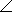# Online Electrical Engineering Test - Electrical Engineering Test 7Loading Test...

Instruction:

• This is a FREE online test. DO NOT pay money to anyone to attend this test.
• Total number of questions : 20.
• Time alloted : 30 minutes.
• Each question carry 1 mark, no negative marks.
• DO NOT refresh the page.
• All the best :-).

1.

The number 65,000 expressed in scientific notation as a number between 1 and 10 times a power of ten is

A.
 0.65 × 104B.
 6.5 × 104C.
 65 × 104D.
 650 × 1032.

Current flows in a circuit when

A.
 a switch is openedB.
 a switch is closedC.
 the switch is either open or closedD.
 there is no voltage3.

A potentiometer is a two-terminal variable resistor.

A.
 TrueB.
 False4.

Five light bulbs are connected in parallel across 110 V. Each bulb is rated at 200 W. The current through each bulb is approximately

A.
 2.2 AB.
 137 mAC.
 1.8 AD.
 9.09 A5.

A certain Wheatstone bridge has the following resistor values: R1 = 10 k, R2 = 720, and R4 = 2.4 k. The unknown resistance is

A.
 24B.
 2.4C.
 300D.
 3,0006.

An ideal current source has zero internal resistance.

A.
 TrueB.
 False7.

Conversions between delta-type and wye-type circuit arrangements are useful in certain specialized applications.

A.
 TrueB.
 False8.

The voltage induced across a certain coil is 200 mV. A 120resistor is connected to the coil terminals. The induced current is

A.
 1.7 mAB.
 16 mAC.
 12 mAD.
 120 mA9.

A sine wave of 15 kHz is changing at a faster rate than a sine wave with a frequency of

A.
 25 kHzB.
 12 kHzC.
 18 kHzD.
 1.3 MHz10.

Referring to Problem 23, what is the circuit impedance, Z?

A.
 264–55.9°B.
 264–34°C.
 2.64–55.9°D.
 2.64–34°11.

Positive numbers can be represented by points to the right of the origin on the horizontal axis of a graph.

A.
 TrueB.
 False12.

A 47resistor is in series with an inductive reactance of 120across an ac source. The impedance, expressed in polar form, is

A.
 4768.6°B.
 12068.6°C.
 12931.4°D.
 12968.6°13.

Total current in an RL circuit always lags the source voltage.

A.
 TrueB.
 False14.

The impedance of an RL circuit varies inversely with frequency.

A.
 TrueB.
 False15.

Referring to Problem 10, the total current is

A.
 5.3 mAB.
 4.6 AC.
 4.6 mAD.
 31 mA16.

Referring to Problem 8, the total current is

A.
 1.5 AB.
 573 mAC.
 5.73 AD.
 1.19 A17.

If the value of C in a series RLC circuit is decreased, the resonant frequency

A.
 is not affectedB.
 increasesC.
 is reduced to zeroD.
 decreases18.

The roll-off rate of a basic RC or RL filter is 20 dB per decade.

A.
 TrueB.
 False19.

If two currents are in the same direction at any instant of time in a given branch of a circuit, the net current at that instant

A.
 is zeroB.
 is the sum of the two currentsC.
 is the difference between the two currentsD.
 cannot be determined20.

In a certain Y-Y system, the source phase currents each have a magnitude of 9 A. The magnitude of each load current for a balanced load condition is

A.
 3 AB.
 6 AC.
 9 AD.
 27 A